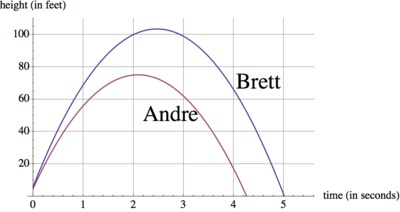# Throwing Baseballs

Alignments to Content Standards: F-IF.B.4 F-IF.C.9

Suppose Brett and Andre each throw a baseball into the air. The height of Brett's baseball is given by $$h(t)=-16t^2+79t+6,$$ where $h$ is in feet and $t$ is in seconds. The height of Andre's baseball is given by the graph below:Brett claims that his baseball went higher than Andre's, and Andre says that his baseball went higher.

1. Who is right?
2. How long is each baseball airborne?
3. Construct a graph of the height of Brett's throw as a function of time on the same set of axes as the graph of Andre's throw (if not done already), and explain how this can confirm your claims to parts (a) and (b).

## IM Commentary

This task could be used for assessment or for practice. It allows the students to compare characteristics of two quadratic functions that are each represented differently, one as the graph of a quadratic function and one written out algebraically. Specifically, we are asking the students to determine which function has the greatest maximum and the greatest non-negative root.

Students are not given specifics on how to compare the maximums and roots of the two functions, and so there are two different solutions. The first solution is done by algebraically determining the vertex of the function $h(t)$ and the roots directly from the given algebraic function, and then comparing those values with the vertex and roots of the given graph. The second solution goes straight into graphing both functions on the same set of axes and comparing them visually. Either method can be used, though the second solution, using graphing, is by far the quicker of the two.

## Solutions

Solution: Algebraically

1. To determine the peak height of Brett's baseball versus Andre's baseball, we wish to compare the maximums of the two functions. Algebraically, there are several ways to approach finding the maximum ($h$-coordinate of the vertex) of Brett's height function, $h(t)$.

We can first rewrite $h(t)$ is vertex form:

\begin{align} h(t)&=-16t^2+79t+6\\ &=-16\left(t^2-\frac{79}{16}t-\frac{6}{16}\right)\\ &=-16\left(t^2-\frac{79}{16}t+\left(\frac{79}{(2)(16)}\right)^2-\left(\frac{79}{(2)(16)}\right)^2-\frac{6}{16}\right)\\ &=-16\left(t^2-\frac{79}{(2)(16)}\right)^2-(-16)\left(\frac{79}{(2)(16)}\right)^2+6\\ &=-16\left(t^2-\frac{79}{(2)(16)}\right)^2+\frac{6625}{64}. \end{align}

So, we see that the vertex of $h(t)$ occurs at $\left(\frac{79}{(2)(16)},\frac{6625}{64}\right)$, and so Brett's baseball reaches a maximum height of $\frac{6625}{64}\approx 103.52$, or approximately 104 feet from the ground.

Note that the vertex occurs at $t=\frac{79}{(2)(16)}=\frac{-79}{(2)(-16)}$, which matches the known formula $t=-\frac{b}{2a}$ where $b$ is the coefficient on $t$ and $a$ is the coefficient on $t^2$. If this formula was already known, we could have found $h(t)$ for this $t$-value instead of converting the function into vertex form: $$h\left(\frac{79}{(2)(32)}\right)=h(2.47)=-16(2.47)^2+79(2.47)+6\approx103.52,$$ or approximately 104 feet above the ground.

We can also find the $t$-coordinate of the vertex by determining the midway point between the two roots of $h(t)$. Setting $h(t)=0$ and using the quadratic formula, we have $$0=-16t^2+79t+6$$ $$\implies t=\frac{-79\pm\sqrt{79^2-4(-16)(6)}}{2(-16)}$$ $$\implies t=\frac{-79\pm\sqrt{6625}}{-32}$$ $$\implies t\approx 5.01 \mbox{ and } t\approx -0.07$$ To find the midway point between these two numbers, we average them. $$\frac{5.01+(-0.07)}{2}=2.47$$ This is the same $t$-coordinate we found above, and similarly we evaluate $h(2.47)$ to find a maximum height of Brett's throw at approximately 104 feet.

Looking at the graph of the height of Andre's baseball, we see that his throw does not even get higher than 80 feet off the ground. So, Brett's baseball went higher.
2. We know that each boy let go of his baseball at $t=0$ seconds. To determine how long each ball was airborne, we need to find the number of seconds, or the $t$-coordinates, when the height of the ball, $h(t)$, is 0.

For Brett, we set his function equal to 0 and determine values of $t$ that make it true. To do this, (if not already done in part (a)) we can use the quadratic formula. $$0=-16t^2+79t+6$$ $$\implies t=\frac{-79\pm\sqrt{79^2-4(-16)(6)}}{2(-16)}$$ $$\implies t=\frac{-79\pm\sqrt{6625}}{-32}$$ $$\implies t\approx 5.01 \mbox{ and } t\approx -0.07$$ Interpreting these two answers, this means that Brett's ball has a height of 0 feet at approximately $-0.07$ and at $5.01$ seconds. Negative seconds do not make sense in our situation, as when Brett first threw the ball is considered 0 seconds, and so we see that Brett's baseball was airborne for about 5 seconds.

Looking at Andre's graph, we see that the ball left his hands at $t=0$, and then returned to the ground (i.e. had a height of 0) at approximately $t=4.2$. So, Andre's baseball was airborne for about 4.2 seconds.

3. By graphing Brett's height function on the same axes as Andre's height, we arrive at the following graph:Here, we can clearly see that Brett's throw went higher, as his graph reaches a greater $h$-value, and that his ball is airborne for longer, as his graph "touches down" on the $t$-axis after Andre's. This supports the conclusions we came to in parts (a) and (b).

Solution: Graphically

1. Alternatively, we can determine which function has the greatest maximum by graphing $h(t)$ on the same set of axes as the graph of Andre's height function, and visually comparing the heights of the vertices.Clearly, we can see here that Brett's throw reached a maximum height of over 100 feet, while Andre's throw did not reach above 80 feet. Therefore, Brett's baseball went higher.

2. Using our graph, we can interpret "how long has the baseball been airborne?" as "how many seconds until the graph crosses the $t$-axis?" Both boys let go of their baseballs at $t=0$ seconds. Andre's graph touches the $t$-axis next at about 4.2, so his baseball was airborne for about 4.2 seconds. Brett's graph touches the $t$-axis at about 5, so his baseball was airborne for about 5 seconds.

3. We have worked through this problem by graphing, and so our graph, of course, supports our conclusions.